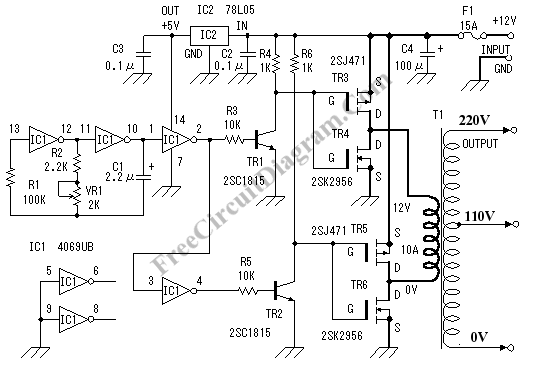# DC-AC Inverter, Convert 12V DC Voltage to 110/220V AC VoltageThe DC to AC inverter circuit shown in the schematic here is very simple circuit since it produce square wave, but it’s enough to power many devices. Here is the schematic diagram of the inverter circuit:The MOSFET is configured as bridge, so the current will flow alternatively in through the primary windings of the transformer. The most expensive part of this dc-ac inverter circuit is the transformer, since it must handle high current up to 10 Amps. You can power any device up to 120 Watts using this 12V inverter circuit.